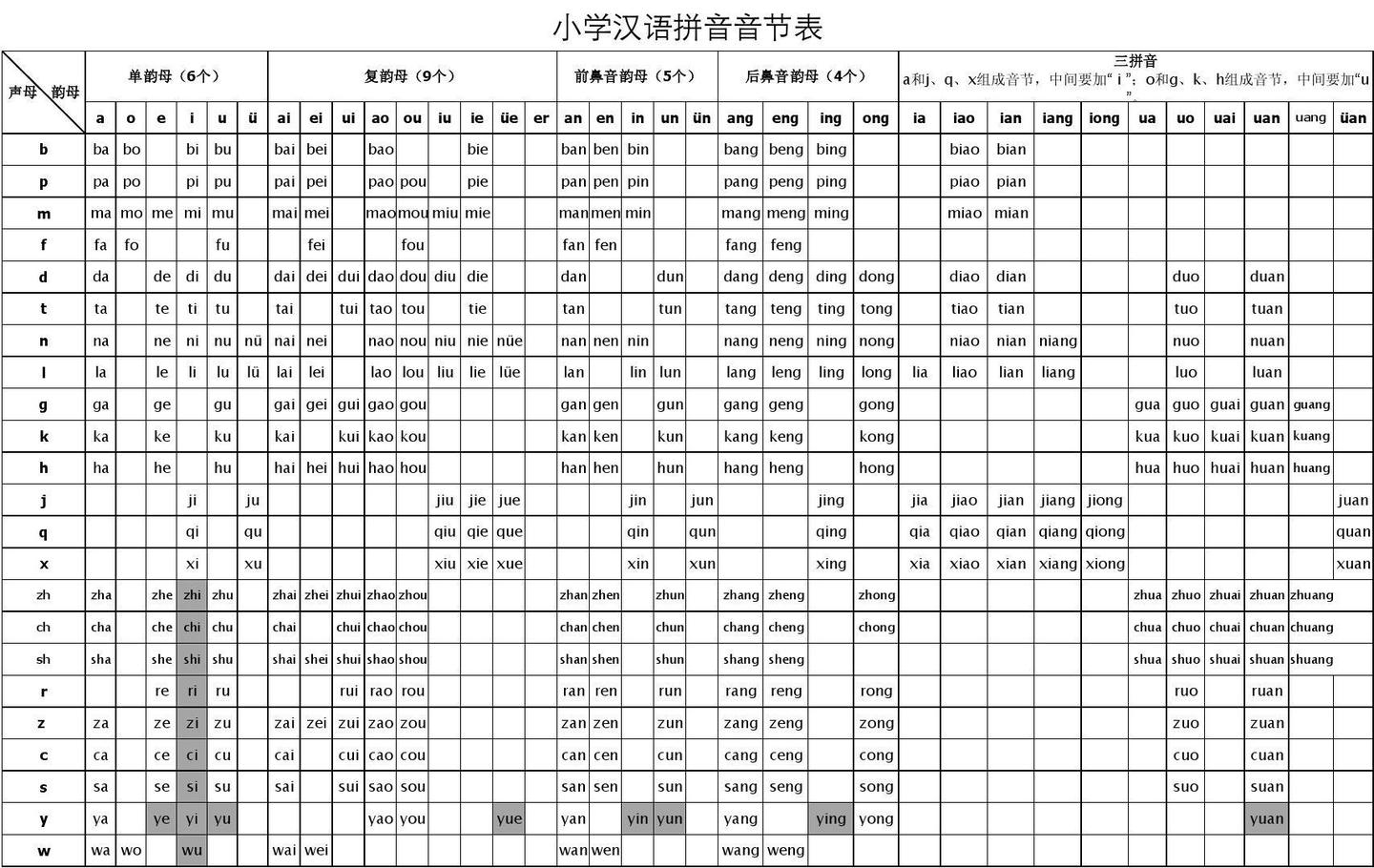## 实现原理

``````let name = '网易杭州';
let sortedName = name.split('').sort((a, b) => {
// 如果我没记错的话，早期的这个方法并不需要第二个参数
return a.localeCompare(b, 'zh-CN');
}).join('');
console.log(sortedName); // 输出 '杭网易州'
``````

## 汉字拼音映射表

``````let start = 0x4e00, end = 0x9FA5;
let result = {};
let get = function () {
if (start > end) {
// 此时 result 就包含了所有的汉字以及相应的拼音
console.log(JSON.stringify(result));
return;
}
let arr = []
for (let i = start; i < start + 3000; i++) {
if (i <= end) {
arr.push(String.fromCharCode(i))
}
}
let xhr = new XMLHttpRequest();
let fd = new FormData();
fd.append('zwzyp_zhongwen', arr.join(''));
fd.append('zwzyp_shengdiao', 0);
fd.append('zwzyp_wenzi', 1);
fd.append('zwzyp_jiange', 1);
fd.append('zwzyp_duozhongduyin', 0);
let div = document.createElement('div')
div.innerHTML = evt.target.responseText;
let pinyins = div.querySelectorAll('.xiaokuang_py');
let hanzis = div.querySelectorAll('.xiaokuang_z');
for(let i = 0; i < hanzis.length; i++) {
result[hanzis[i].innerHTML] = pinyins[i].innerHTML;
}
start += 3000;
get();
}
xhr.open('POST','https://zhongwenzhuanpinyin.51240.com/web_system/51240_com_www/system/file/zhongwenzhuanpinyin/data/');
xhr.send(fd);
}
get();
``````

``````// 此表可以根据自身需求进行删减
let fixedHanzis = {
'沈': 'shen', '嗲': 'dia', '碡': 'zhou', '聒': 'guo', '炔': 'que',
'蚵': 'ke', '砉': 'hua', '嬤': 'mo', '蹒': 'pan', '丬': 'pan', '霰': 'xian',
'豉': 'chi', '饧': 'xing', '帧': 'zhen', '郍': 'na', '芎': 'xiong', '谁': 'shui'
}
``````

## 具体实现

``````var COLLATOR = new Intl.Collator(['zh-CN'])

COLLATOR.compare('网', '易') // 输出 -1
``````

## 吐槽

• 有些多音字选择了不常见的读音，比如“沈”，在 Chrome 中是“chen”，而不是常见的“shen”，又比如“呵”，在 Safari 中念“a”
• 读字读半边，这种例子有很多，比如“碡”，念成了“du”（正确的读音是 zhóu），“聒”念成了“gua”（正确的读音是 guō）
• 纯粹乱读，Safari 中有很多，比如“鼰”，念成了“nian”（正确的读音是 jú），“罉”，念成了“cang”（正确的读音是 chēng），“伝”，念成了“chuan”（正确的读音是 yún），“枡”，念成了“dou”（正确的读音是 shēng）

## 参考资料

• 本方案并非原创，而是参考了 pinyin 的实现，并在它的基础做了一点微小的工作。如文中所说，该方案是从 Android 源码中扒出来的。